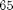## Loren on the Art of MATLABTurn ideas into MATLAB

Note

Loren on the Art of MATLAB has been archived and will not be updated.

# Rounding Results

There are frequent questions on the MATLAB newsgroup about rounding results to a certain number of decimal places. MATLAB itself doesn't provide this functionality explicitly, though it is easy to accomplish.

### Sidetrack : A Little MathWorks History

MathWorks' first Massachusetts office phone number was 653-1415 (ignoring country and area codes). The astute reader will notice that the last 5 digits are an approximation for(or, in MATLAB, pi). A local resident called one day to say that she kept getting calls for MathWorks and she wasn't sure why. But it was quite inconvenient for her because she spent lots of time on the second floor of her home, and the phone was on the first floor. The excess round-trips were taxing her! To understand what was happening, you should know that in some of the early MathWorks materials, the phone number was listed as.

### Example

format long
x = 1.23456789
x =
1.234567890000000


Use round. Here we get no decimals at all.

round(x)
ans =
1


There are many ways to get the number of decimals you want. Here's one way to round to 3 decimals.

round(x*1000)/1000
ans =
1.235000000000000


Here's another way.

sprintf('%0.3f',x)
ans =
1.235


And another. In this case, using the "easy" way to specify the format, you need to know how many integral digits there are as well.

str2num(num2str(x,4))
ans =
1.235000000000000


### Tools for Rounding Solutions

There are a lot of tools for helping you round numbers. The functions I list here for MATLAB form the basis of many, if not all, of the specific solutions.

MATLAB

Mapping Toolbox

File Exchange Rounding Tools

% N= num2str(X,SF);
% N= str2num(N);

### Question for You

When you round values, do you want this for display only, or wanted for calculations? Some applications I can think of might include processing data that has a smaller number of bits of precision to start with. What applications do you need rounding for in your calculations? Post here with your thoughts.

Published with MATLAB® 7.8

### 댓글

댓글을 남기려면 링크 를 클릭하여 MathWorks 계정에 로그인하거나 계정을 새로 만드십시오.